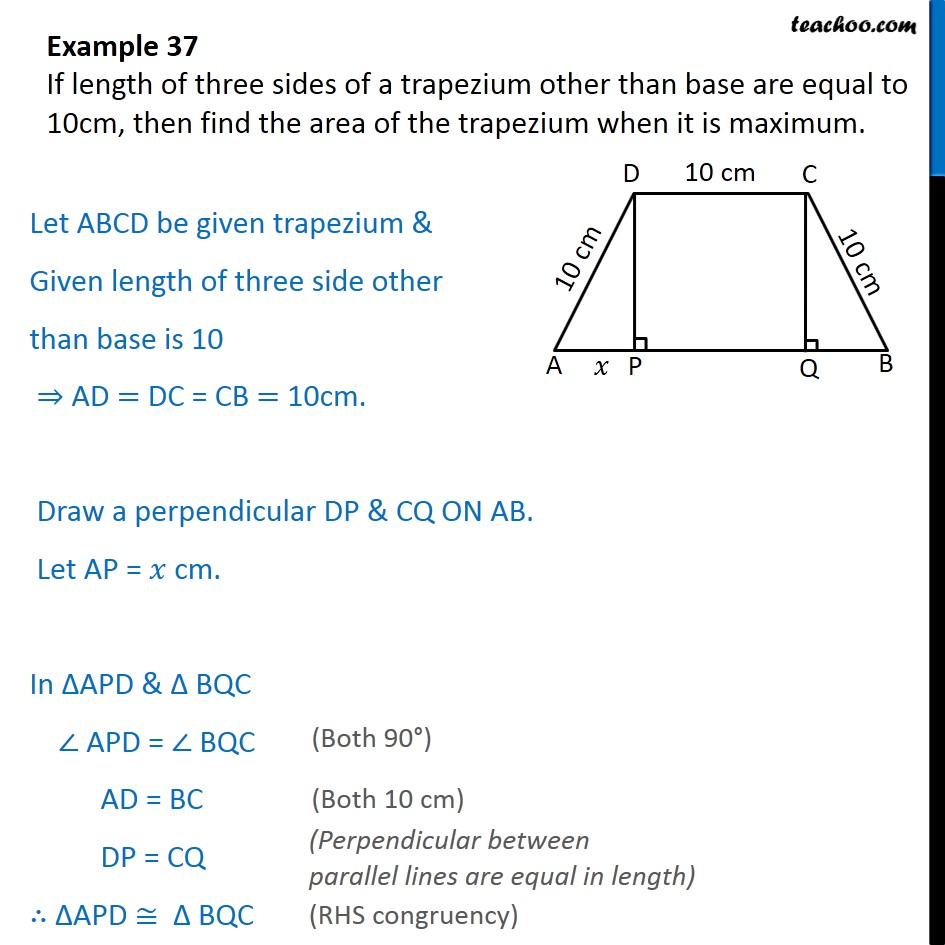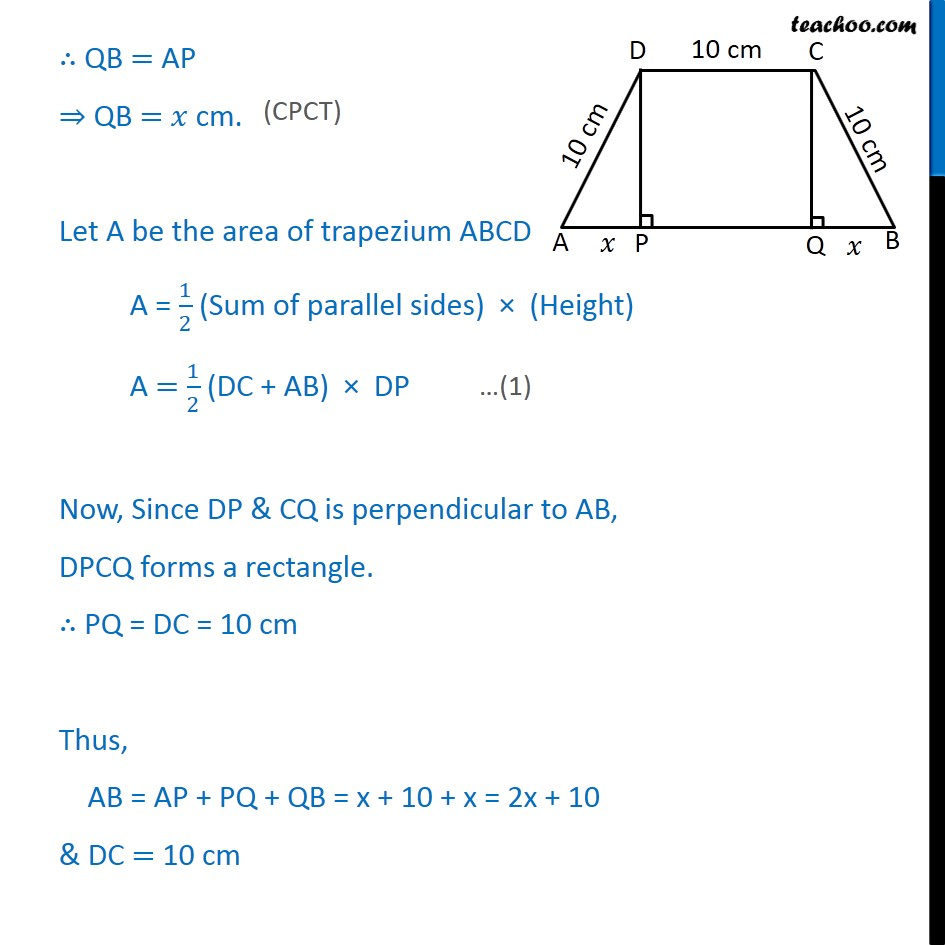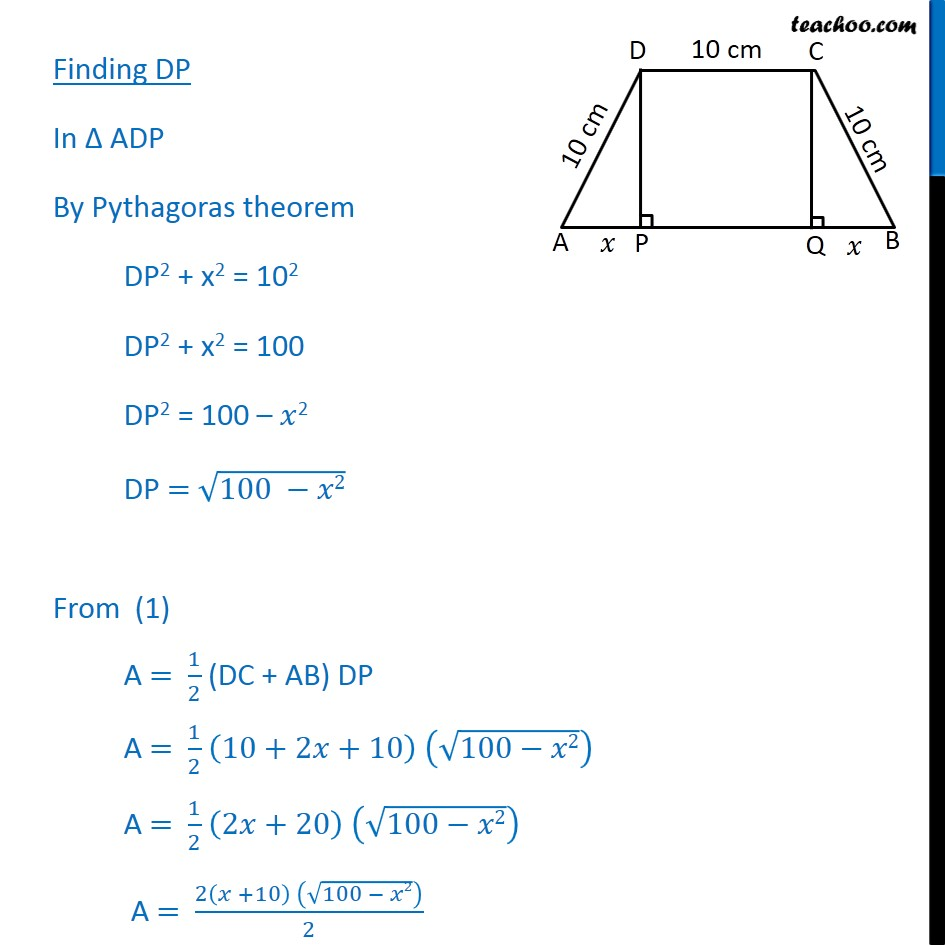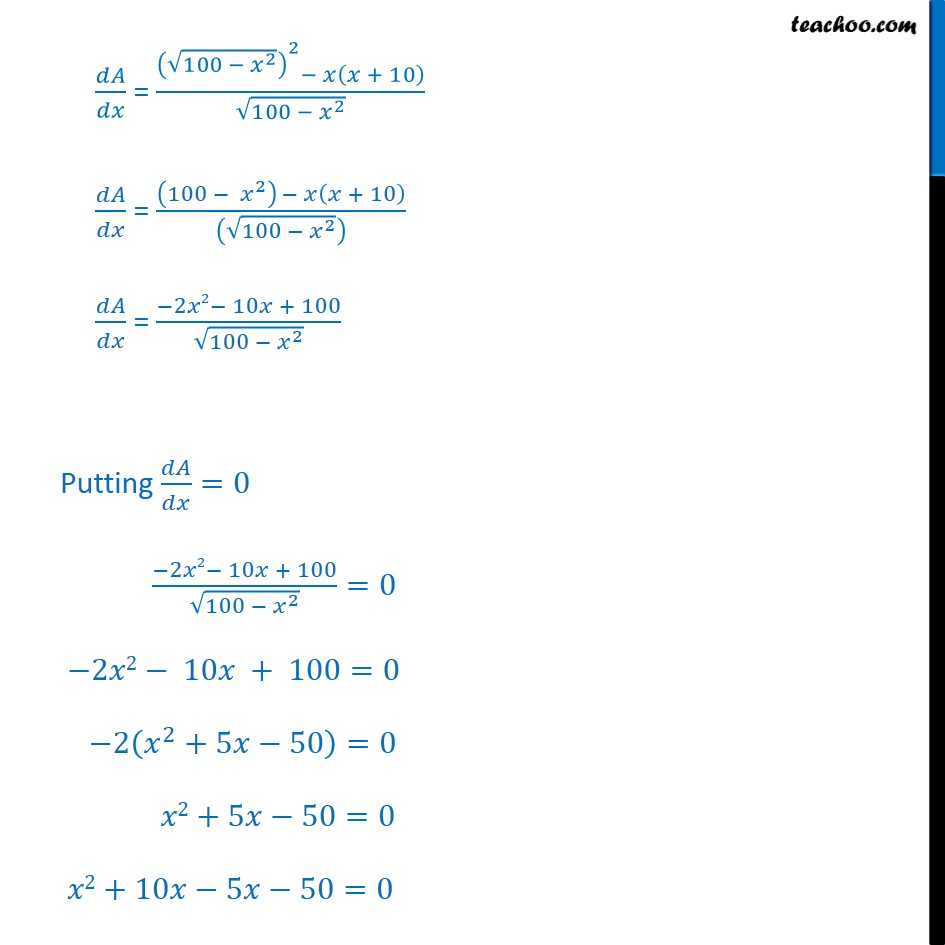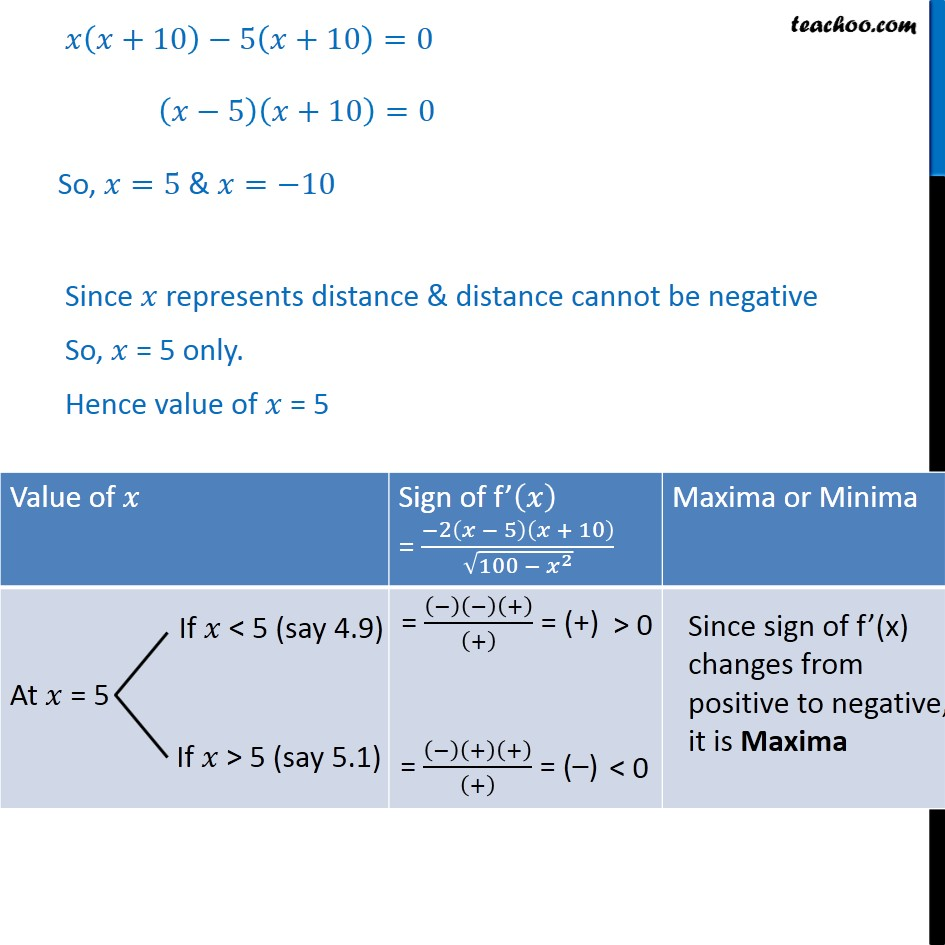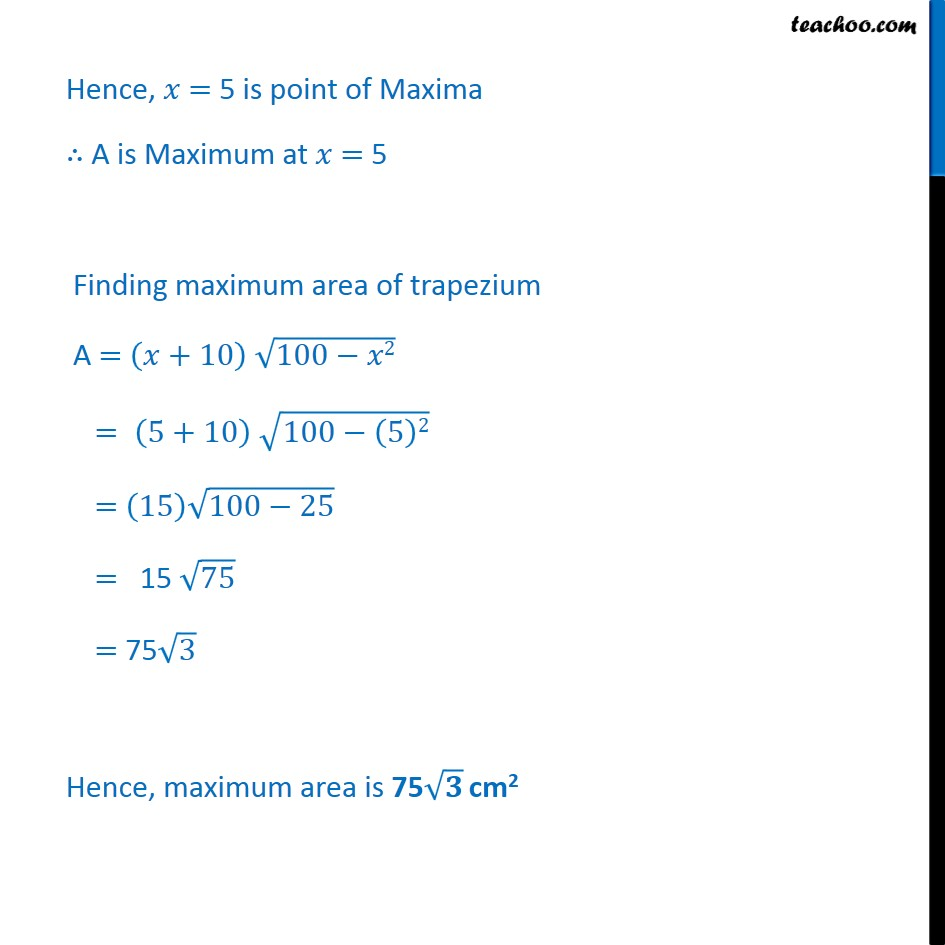1. Class 12
2. Important Question for exams Class 12
3. Chapter 6 Class 12 Application of Derivatives

Transcript

Example 37 If length of three sides of a trapezium other than base are equal to 10cm, then find the area of the trapezium when it is maximum. Let ABCD be given trapezium & Given length of three side other than base is 10 AD = DC = CB = 10cm. Draw a perpendicular DP & CQ ON AB. Let AP = cm. In APD & BQC APD = BQC AD = BC DP = CQ APD BQC QB = AP QB = cm. Let A be the area of trapezium ABCD A = 1 2 (Sum of parallel sides) (Height) A = 1 2 (DC + AB) DP Now, Since DP & CQ is perpendicular to AB, DPCQ forms a rectangle. PQ = DC = 10 cm Thus, AB = AP + PQ + QB = x + 10 + x = 2x + 10 & DC = 10 cm Finding DP In ADP By Pythagoras theorem DP2 + x2 = 102 DP2 + x2 = 100 DP2 = 100 2 DP = 100 2 From (1) A = 1 2 (DC + AB) DP A = 1 2 10+2 +10 100 2 A = 1 2 2 +20 100 2 A = 2 +10 100 2 2 A = +10 100 2 We need to find area of trapezium it is maximum i.e. we need to Maximize Area A = +10 100 2 Diff w.r.t = + 10 100 2 = 10 . 100 2 + 100 2 . +10 = 1+0 100 2 + 1 2 100 2 . 100 2 . +10 = 100 2 + 1 2 100 2 . 0 2 +10 = 100 2 2 + 10 2 100 2 = 100 2 2 + 10 100 2 = 100 2 + 10 100 2 = 2 2 10 + 100 100 2 Putting =0 2 2 10 + 100 100 2 =0 2 2 10 + 100=0 2 2 +5 50 =0 2+5 50=0 2+10 5 50=0 +10 5 +10 =0 5 +10 =0 So, =5 & = 10 Since represents distance & distance cannot be negative So, = 5 only. Hence value of = 5 Hence, = 5 is point of Maxima A is Maximum at = 5 Finding maximum area of trapezium A = +10 100 2 = 5+10 100 5 2 = 15 100 25 = 15 75 = 75 3 Hence, maximum area is 75 cm2

Chapter 6 Class 12 Application of Derivatives

Class 12
Important Question for exams Class 12